# How to Convert Weekly Data into Monthly Data in ExcelData comes to us in many forms, and often our biggest challenge is translating it from the form it came in into the form we need it in. Date-based data is especially challenging – there are days of the week, weekly totals, months with different numbers of days, and holidays that land on different weekdays each year. This article is part of a series that will help you work with date-based data in Excel to get it into the formats you need.

This tutorial will teach you how to convert weekly summary data into monthly total data by allocating the days in each week to the appropriate month of the year. Let’s dive in!

## Examine the Data Set

First off, let’s take a look at our sample data…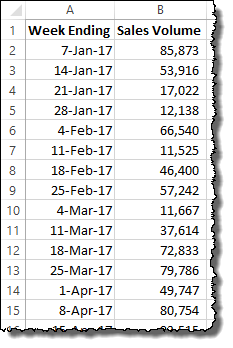Here we have some pretty standard weekly aggregated sales data. In Column A, we have a date field listing the ending date of each week (Saturday). In Column B we have total sales that occurred in that week.

Throughout this tutorial, we are going to assume that the date provided in your weekly data is the week end. It is still possible to convert week beginning dates and data, but the formulas will need to be changed.

## Conversion Assumptions

Since we have only summarized data about each week, there is no way to know exactly what each day’s data was, so you will need to make some assumptions about the daily data. In this tutorial, we’ll assume that each day is average – 1/7th of the week’s total. You could make a different assumption, but it would make the formulas more complicated.

To allocate the correct number of days to each month, we need to build a formula that counts how many days in the week were in the prior month and how many were in the current month. Most weeks will be fully in the current month, so that month will get all of the days. To do this clearly, we are going to create a helper column that helps us calculate the conversion.

## Making the Helper Column

In the column next to your weekly data (in our case Column C), create new header and call it “Days In Month” or something similar (the title is just for reference). In the row with the first line of data, type the following formula and press ENTER: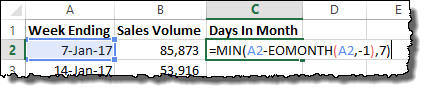`=MIN(A2-EOMONTH(A2,-1),7)`

This formula uses the EOMONTH() function. This function provides the date value for the last day of any month, relative to the date provided (The End Of the MONTH). The syntax for the EOMONTH() function is as follows:

`EOMONTH(start_date, months)`

The start_date is the reference date in Excel date format. The months input is any integer number, positive or negative. If months is zero (0), EOMONTH() provides the last date of the start_date month. If 1, the last day of the next month. If -1, the last day of the prior month, and so on.

In this example, EOMONTH(A2,-1) returns the last day of the month prior to the date in Column A.

The MIN() function just returns the lower of two numbers. In this example, it will calculate (A2EOMONTH(A2,-1)) and return whatever is lower – the answer, or the number 7.

In cell C2, The formula is calculating as follows:

```MIN(7-Jan-17 - 31-Dec-16 = 7, 7)
```

In this case, the answers are the same, so the MIN() function returns 7.

Drag the formula down to copy it to all the rows in your data set. When you are finished, you should have a table of data like this: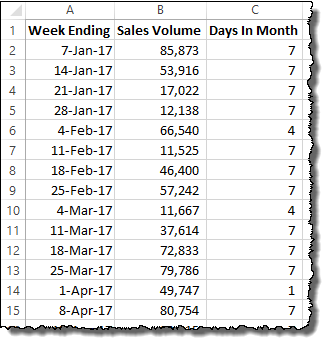Now, we can add up all the parts of the month to get our monthly totals…

## Building the Monthly Total Formula, Part 1

To begin, we need to set up a monthly table.  Next to your data table, build a row of month calendar headings using dates, like shown: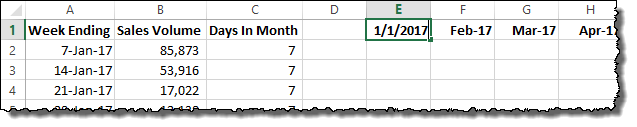Now, we can begin to capture the portion of the data that falls into each month. To begin, type the following formula in cell E2 and press ENTER:

```=SUMPRODUCT((DATE(YEAR(\$A\$2:\$A\$54),MONTH(\$A\$2:\$A\$54),1)=DATE(YEAR(E\$1),
MONTH(E\$1),1))*\$C\$2:\$C\$54/7*(\$B\$2:\$B\$54))```

It will look like below, and don’t worry – we’ll walk through what’s going on: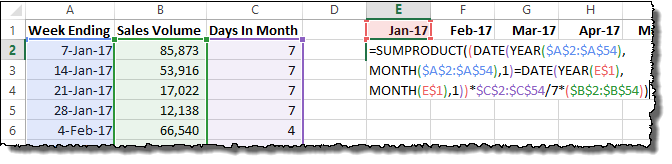The SUMPRODUCT() function is one big multiplication calculation, but it doing that multiplication for every row in the data table. That’s what the ranges are for (\$A\$2:\$A\$54\$B\$2:\$B\$54, and \$C\$2:\$C\$54). The part with the DATE() functions is actually comparing two dates and returning a 1 if they are equal and a 0 if they are inequal. Let’s look closer:

```(DATE(YEAR(\$A\$2:\$A\$54),MONTH(\$A\$2:\$A\$54),1) = DATE(YEAR(E\$1),
MONTH(E\$1),1))```

The first DATE() function is building an Excel date value equal to the month and year of every row in the data table. The second DATE() function is building an Excel date value equal to the month and year of the monthly calendar table.

If they are equal, the SUMPRODUCT() will include the row in the sum. Otherwise, the formula will add zero (excluding them from the sum).

For every row with a date in the current month/year, the rest of the formula is considered:

`\$C\$2:\$C\$54/7*(\$B\$2:\$B\$54)`

Since Column C is our helper column, it contains the number of days that count in the current month. That number, divided by 7, gives us the fraction of the weekly total to allocate to the current month’s total. We multiply that by the total in Column B, and that’s it!

The SUMPRODUCT() takes the sum of all rows with partial weeks in the current month and adds them together to get the first part of our monthly total.

## Building the Monthly Total Formula, Part 2

The first SUMPRODUCT() formula got most of the values we needed, but it left out the partial months that ended mid-week. For example, the week ending February 4, 2017 has 3 days of January in it! To add these days to the total, we need to build another SUMPRODUCT() formula…

Type the following formula in cell E3 and press ENTER:

```=SUMPRODUCT((DATE(YEAR(\$A\$2:\$A\$54),MONTH(\$A\$2:\$A\$54),1)=DATE(YEAR(E\$1),
MONTH(E\$1)+1,1))*(7-\$C\$2:\$C\$54)/7*(\$B\$2:\$B\$54))```

It will look like below: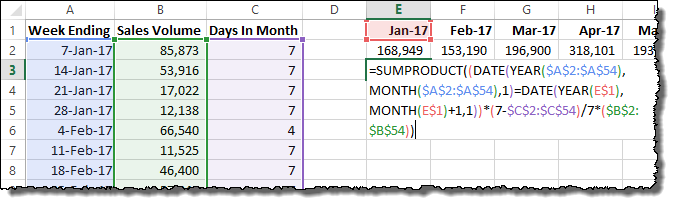The same logic is at work here as in the previous formula with a few minor tweaks. Instead of comparing dates in Column A to the current month in the monthly calendar table, the formula compares it to the next month in the calendar table:

```(DATE(YEAR(\$A\$2:\$A\$54),MONTH(\$A\$2:\$A\$54),1)=DATE(YEAR(E\$1),
MONTH(E\$1)+1,1))```

The only difference is a small +1 added to the MONTH() portion of the DATE() formula, highlighted here in red.

The rest of the SUMPRODUCT() formula has also changed:

`(7-\$C\$2:\$C\$54)/7*(\$B\$2:\$B\$54)`

Instead of using helper Column C as the fraction, we are taking the opposite fraction. Since 7 is our denominator, we start with 7 and subtract the number of days in the (next) month to get the remainder. That fraction of the week is multiplied by the weekly total data and then added to our SUMPRODUCT() total.

## Combining the Monthly Total Formulas

Fortunately, these two SUMPRODUCT() functions can be added together in a single cell to create a total monthly figure for each of the monthly calendar columns. It is as simple as pasting the formulas together with a + in the middle:

```=SUMPRODUCT((DATE(YEAR(\$A\$2:\$A\$54),MONTH(\$A\$2:\$A\$54),1)=DATE(YEAR(E\$1),
MONTH(E\$1),1))*\$C\$2:\$C\$54/7*(\$B\$2:\$B\$54)) +
SUMPRODUCT((DATE(YEAR(\$A\$2:\$A\$54),MONTH(\$A\$2:\$A\$54),1)=DATE(YEAR(E\$1),
MONTH(E\$1)+1,1))*(7-\$C\$2:\$C\$54)/7*(\$B\$2:\$B\$54))```

It will look like below when completed: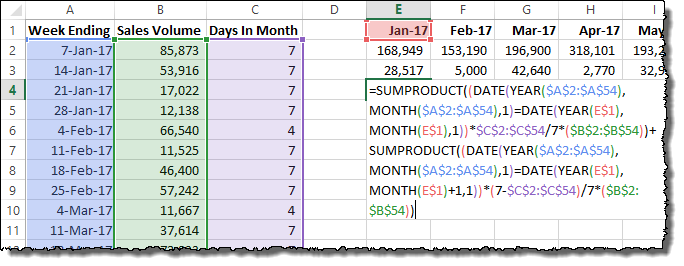You can drag all three formulas to the right to copy them to each monthly calendar table column. At the end, you should have the same total amount across all your months as you have in your original weekly data set. You can delete the first two rows of the calendar if you prefer, leaving only the combined total monthly sums.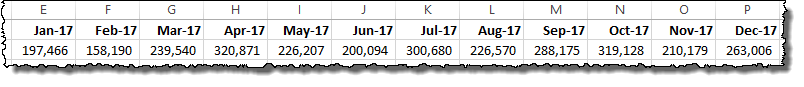The example data used here, as well as all of the formulas discussed is available for download below. You can experiment with the example data in the embed, or download the entire Excel spreadsheet by clicking the download in the bottom right.

## Get the latest Excel tips and tricks by joining the newsletter!Andrew Roberts has been solving business problems with Microsoft Excel for over a decade. Excel Tactics is dedicated to helping you master it.

Join the newsletter to stay on top of the latest articles. Sign up and you'll get a free guide with 10 time-saving keyboard shortcuts!

## 15 thoughts on “How to Convert Weekly Data into Monthly Data in Excel”

1. O says:

Hi there – this formula is great!

I was wondering if you had a solution to a modification I need to make to this.

I have a large volume of date that has weekly data for about a few hundred individual sites.

I have used this formula to calculate monthly totals for all sites, but I was wondering how I would go about using to calculate monthly totals for each individual site, without having to separate out all the data?

Any tips would be really useful!

Thanks!

1. Josiah says:

I believe I have the same or similar query. I have multiple people making sales, and so need the array B2:B54 in the example to be dynamic based on the header — so if Person 1 was B1 and Person 2 was C1, the formula would use B2:54 if B1 matched “Person 1” and would use C2:C54 if C1 matched “Person 2” as I’m trying to find their sales for the month.

Hii, Firstly thanks for providing the solution.
Can we apply the above procedure for meteorological parameters as( Raianfall, Temperature,etc)?

3. Joe Iocona says:

How would you do this to account for work days only (M-F) not averaging Saturday and Sunday?

1. R MacDougall says:

To just prorate over net workdays, you need to modify the formula for “Days in Month” and add a column for net workdays in the week which replaces the 7 days used in the formulas. In the NETWORKDAYS function I use a named range “holidays” which is a list of holidays for the period.

In column C, change =MIN(B2-EOMONTH(B2,-1),7) to =MIN(NETWORKDAYS(EOMONTH(B2,-1)+1,B2,holidays),NETWORKDAYS(B2-7,B2,holidays))
This takes the smaller of (1) net workdays from the beginning of the month or (2) of the net work days in the week ending B2.

Add in column D the total net workdays in the week ending on B2
=NETWORKDAYS(B2-7,B2,holidays)

Then replace the 7 used in the SUMPRODUCT functions with \$D\$2:\$D\$54

The new formula is:
=SUMPRODUCT((DATE(YEAR(\$A\$2:\$A\$54),MONTH(\$A\$2:\$A\$54),1)=DATE(YEAR(E\$1),MONTH(E\$1),1))*\$C\$2:\$C\$54/\$D\$2:\$D\$54*(\$B\$2:\$B\$54))+SUMPRODUCT((DATE(YEAR(\$A\$2:\$A\$54),MONTH(\$A\$2:\$A\$54),1)=DATE(YEAR(E\$1),
MONTH(E\$1)+1,1))*(\$D\$2:\$D\$54-\$C\$2:\$C\$54)/\$D\$2:\$D\$54*(\$B\$2:\$B\$54))

4. Tugce says:

Is there any suggestion how to convert monthly data to weekly data?

5. Brian Vandenberg says:

This is excellent! Thank you

6. David Simon says:

Really great approach, thank you so much for sharing, works great!

7. Abby Larreau says:

How do the formulas have to be changed if I want to calculate with week beginning on Monday? Can you please follow up and let me know? Thank you!

8. Pavan says:

I don’t comment too often but this solution is brilliant. Love the approach.

9. Mark Jones says:

My data has item, customer, and week, so the sumproduct formula needed to factor in 3 criteria instead of 1…not a problem except that with 200,000 rows excel kept crashing and even when it didn’t, the calculation time was very inconvenient. So I needed a way that avoids and lookup method. I found other methods using queries instead of lookups, but it involves writing a list of possible combinations and the query language was complex and foreign to me. So what I arrived at was four additional helper columns, 1) the current month, 2) the units allocated to that month (by a percentage of the days vs. 7), 3) the previous month, 4) the units allocated to that month by the leftover percentage in a partial week situation.

Then the problem was that I had two different month fields and two different quantity fields to combine. I created one query that looked only at the current month/quantity (first two of the new helper columns) and another query that looked only the previous month/quantity (last two of the new helper columns). I renamed the Month and the Units to a generic “Month” and “Monthly Units” in each of those queries, and did a additional append query combining the two together. Now I can pivot that data and it all works out. Best of the queries run very fast and it can handle the large data with multiple criteria. There’s probably a way to do this all in one query but I’m not that advanced!

10. Ken Carter says:

Similar. I have Data that (OEM EDI 830 forecast) which comes in in Weekly Buckets with Week stating on Mondays thru Sunday. The volumes given are the total units required for the given week. I need to convert these weekly volumes into calendar monthly volumes, and account for the proper monthly splits when month ends mid week. Any assistance with creating this modified formula would be A GREAT HELP! Thank you.

Data. Week 43 10/24//22 500 each
Week 44 10/31/22 600 each
Week 45 11/7/22 200 each etc.

11. Tycho G. says:

Seems like this isn’t working anymore, at least on my end. I try to replicate this on Excel and for the entries in the helper column, i’m getting:
1/7/1900
1/7/1900
1/7/1900
1/7/1900
1/4/1900
1/7/1900
1/7/1900
1/7/1900
1/3/1900
1/7/1900
1/7/1900
1/7/1900
1/7/1900
1/7/1900
1/7/1900
1/7/1900
1/7/1900
1/5/1900
1/7/1900

and so on. Has anyone been able to find a solution to this problem?

12. Sam says:

This DOES NOT WORK, you miss the end of the month where the last week of data isn’t the very end of the month.

13. Derek says:

That column needs to be a number field not a date field